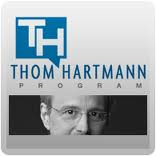On July 23, 2016, we discontinued our forums. We ask our members to please join us in our new community site, The Hartmann Report. Please note that you will have to register a new account on The Hartmann Report.

2 posts / 0 new

/the-magic-of-math-arthur-benjamin_aka mathemagician

Whatever number you have, subtract or add single digits to the closest multiple of 10 Ex: 12 subtract 2 to reach 10, anyone can do multiples of 10 in their head. Now add to the original number the same amount you subtracted, 12 + 2 = 14, 10 x 14 is 140. Now go to the number you subtracted and added, in this case 2, and square it 2x2=4, add 4 to your first sum, 144 is the square of 12 (but we all knew that anyhow) 96 squared means adding 4 to get to a 100, subtracting 4 to 92, 100 x 92 = 9200. Square the 4 that you added and subtracted.. 4 x 4 =16 96 squared is 9216

btw, you could do the 12 square by adding 8 to get to 20, subtracting 8 to get to 4,

4 x 20 =80 square the 8 for 64, 80+ 64 =144. Just get to the closest 10 multiple whether it's 20, 50, 100douglaslee
Joined:
Jul. 31, 2007 3:01 pm

46 squared: add 4 for 50, subtract 4 for 42, 50 x 42 = 2100*, 4x4=16, 2116 is the square of 46,

*100 times 42 is 4200, half is 2100douglaslee
Joined:
Jul. 31, 2007 3:01 pm

## Lev Parnas is afraid of Bill Barr - and he should beLev Parnas recently told Rachel Maddow that he's more afraid of Attorney General Bill Barr than he is of the mobbed-up foreign oligarchs he has betrayed. Barr, after all, can weaponize our prisons to punish Parnas.